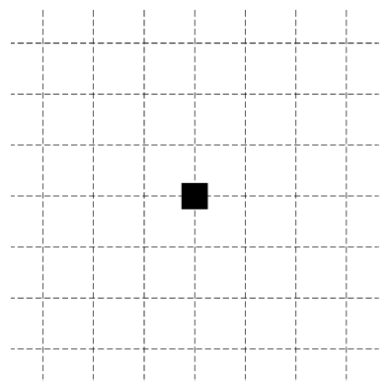# 2017 AP Physics 2物理Algebra-Based真题系列之Practice Exam简答题免费下载

### 真题与答案下载### 2017 AP 物理2模考简答题部分免费下载

#### 部分真题预览：

1）A team of engineering students is testing their newly designed 200kg raft in the pool where the diving team practices. The raft must hold a 730 kg steel cube with edges of length 45.0 cm without sinking. Assume the density of water in the pool is 1000 kg/m3 .

1. The students use a crane to gently place the cube on the raft but accidentally place it off center. The cube remains on the raft for a few moments and then the raft tilts, causing the cube to slide off and sink to the bottom of the pool. The raft remains floating in the pool. In a coherent paragraph-length response, indicate whether the water level in the pool when the cube is on the bottom of the pool is higher than, lower than, or the same as when the cube is on the raft, and explain your reasoning. For both cases, assume that there is no
motion of the water.
2. The bottom of the pool is 5 m below the surface of the water. The crane is now used to lift the cube out of the pool. The cube is lifted upward at a slow and constant speed, so all drag forces are negligible.
1. Predict how the force exerted by the crane on the cube when the bottom of the cube is at a depth of 2.0 m compares to the force exerted by the crane on the cube when the bottom of the cube is at a depth of 4.0 m. Explain your reasoning.
2. On the black square below, which represents the cube, draw and label the forces (not components) that are exerted on the cube while the crane is lifting it and the bottom of the cube is 4 m below the surface of the water. Each force must be represented by a distinct arrow starting on, and pointing away from, the black square. The lengths of the arrows should approximately demonstrate the net force on the cube.3. Calculate the force exerted by the crane on the cube when the bottom of the cube is 4 m below the surface of the water.

### 2017 AP 物理2简答题免费下载